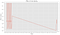# Classification vs Regression

`# Import relevant itemsimport pandas as pdimport numpy as npimport seaborn as snsimport matplotlib.pyplot as pltplt.style.use('ggplot')%matplotlib inlineimport datetime as dtfrom datetime import datetimeimport math# Import CSV file into a dataframedf = pd.read_csv('weather-and-aqi-v5.csv')`

# Cleaning the Data

`#drop unneeded featuresdf = df.drop(['Year', 'Day', 'Date Key', 'Date Key.1', 'AQI', 'Source.Name', 'Site', 'Parameter', 'Unit', 'Duration', 'USAF', 'WBAN', 'GUS', 'CLG', 'SKC', 'L', 'M', 'H', 'VSB', 'MW', 'MW_1', 'MW_2', 'MW_3', 'AW', 'AW_4', 'AW_5', 'AW_6', 'W', 'SLP', 'ALT', 'STP', 'MAX', 'MIN', 'PCP01', 'PCP06', 'PCP24', 'PCPXX', 'SD'], axis=1)`
`df['Date (LST)'] = pd.to_datetime(df['Date (LST)'])df = df.rename(columns={"Date (LST)": "Date"})`
`df['Value_1'] = df.Value.shift(periods=1)df['TEMP_1'] = df.TEMP.shift(periods=1)df['SPD_1'] = df.SPD.shift(periods=1)df['DEWP_1'] = df.DEWP.shift(periods=1)df['DIR_1'] = df.DIR.shift(periods=1)`
`df = df[df.Value <= .7]`This time series goes from 10–01–2015 to 10–20–2015. Clearly these look to be in error.
`df = df[df.Date > '2015-10-20 01']`
`# Show rows where any cell has a NaNdf[df.isnull().any(axis=1)].shapeOutput: (11818, 33)`
`# Shape of dataframedf.shapeOutput: (18583, 33)# Drop rows that contain NaNdf = df.dropna(axis=0)# Shape of dataframedf.shapeOutput: (6765, 33)`

# Feature Engineering

## Convert Value Field from mg to µg

`# 1 mg = 1,000 µgdf['Value'] = df.Value * 1000df['Value_1'] = df.Value_1 * 1000df['Value_2'] = df.Value_2 * 1000df['Value_3'] = df.Value_3 * 1000df['Value_4'] = df.Value_4 * 1000df['Value_5'] = df.Value_5 * 1000`

## Convert TEMP and DEWP from F to C

`# Formula to convert F to C is: [°C] = ([°F] - 32) × 5/9df['TEMP'] = (df.TEMP - 32) * 5.0/9.0df['TEMP_1'] = (df.TEMP_1 - 32) * 5.0/9.0df['TEMP_2'] = (df.TEMP_2 - 32) * 5.0/9.0df['TEMP_3'] = (df.TEMP_3 - 32) * 5.0/9.0df['TEMP_4'] = (df.TEMP_4 - 32) * 5.0/9.0df['TEMP_5'] = (df.TEMP_5 - 32) * 5.0/9.0# Formula to convert F to C is: [°C] = ([°F] - 32) × 5/9df['DEWP'] = (df.DEWP - 32) * 5.0/9.0df['DEWP_1'] = (df.DEWP_1 - 32) * 5.0/9.0df['DEWP_2'] = (df.DEWP_2 - 32) * 5.0/9.0df['DEWP_3'] = (df.DEWP_3 - 32) * 5.0/9.0df['DEWP_4'] = (df.DEWP_4 - 32) * 5.0/9.0df['DEWP_5'] = (df.DEWP_5 - 32) * 5.0/9.0`

## Convert SPD from Mph to Kph

`# 1 mph = 1.60934 kphdf['SPD'] = df.SPD * 1.60934df['SPD_1'] = df.SPD_1 * 1.60934df['SPD_2'] = df.SPD_2 * 1.60934df['SPD_3'] = df.SPD_3 * 1.60934df['SPD_4'] = df.SPD_4 * 1.60934df['SPD_5'] = df.SPD_5 * 1.60934`

## Convert DEWP to HUM

`df['HUM'] = 100*(np.exp((17.625 * df['DEWP'])/(243.04 + df['DEWP']))/np.exp((17.625 * df['TEMP'])/(243.04 + df['TEMP'])))df['HUM_1'] = 100*(np.exp((17.625 * df['DEWP_1'])/(243.04 + df['DEWP_1']))/np.exp((17.625 * df['TEMP_1'])/(243.04 + df['TEMP_1'])))df['HUM_2'] = 100*(np.exp((17.625 * df['DEWP_2'])/(243.04 + df['DEWP_2']))/np.exp((17.625 * df['TEMP_2'])/(243.04 + df['TEMP_2'])))df['HUM_3'] = 100*(np.exp((17.625 * df['DEWP_3'])/(243.04 + df['DEWP_3']))/np.exp((17.625 * df['TEMP_3'])/(243.04 + df['TEMP_3'])))df['HUM_4'] = 100*(np.exp((17.625 * df['DEWP_4'])/(243.04 + df['DEWP_4']))/np.exp((17.625 * df['TEMP_4'])/(243.04 + df['TEMP_4'])))df['HUM_5'] = 100*(np.exp((17.625 * df['DEWP_5'])/(243.04 + df['DEWP_5']))/np.exp((17.625 * df['TEMP_5'])/(243.04 + df['TEMP_5'])))`

## Create day of the week feature

`df['day_week'] = df['Date'].dt.weekday_name`
`df['day_week_cat'] = df.day_week.astype("category").cat.codes`

# Split into Training and Test Data

`from sklearn.model_selection import train_test_splity = df['Value']X = df.drop(['Value'], axis=1)X_train, X_test, y_train, y_test = train_test_split(X, y, test_size=.3, random_state=1234)`

Written by